Question

# Determine the normal force at a section passing through point C.

Take P = 11 kN.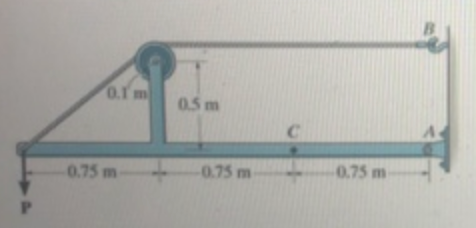Part A

Determine the normal force at a section passing through point C.

Part B

Determine the shear force at a section passing through point C.

Part C.

Determine the moment at a section passing through point C.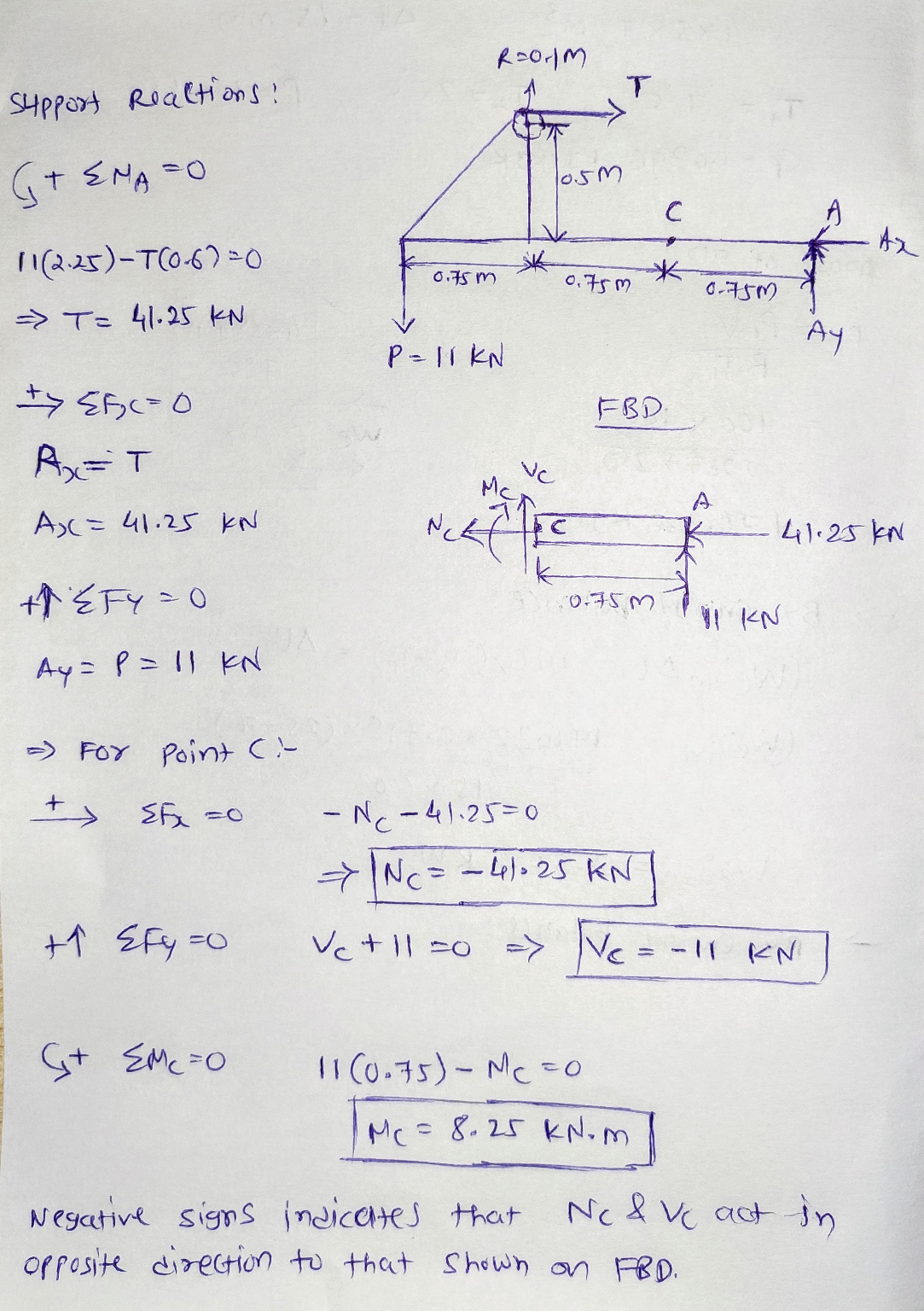#### Earn Coins

Coins can be redeemed for fabulous gifts.

Similar Homework Help Questions
• ### Determine the normal force at section passing through pointD.- Determine the shear force...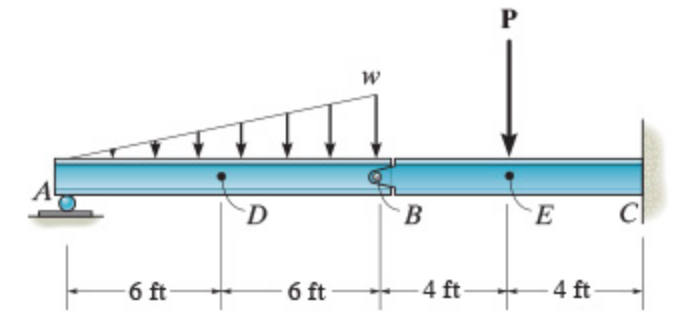- Determine the normal force at section passing through point D.- Determine the shear force at section passing through point D.-Determine the moment at section passing through point D.-Determine the normal force at section passing through point E.-Determine the shear force at section passing through point E.-Determine the moment at section passing through point E.Consider the beam shown in (Figure 1). Suppose P = 6 kip, w = 1.6 kip/ft. Point E is just to the right of the 6-kip...

• ### Determine the normal force, shear force, and moment at a section through point C. Take P = 8 kN.

Determine the normal force, shear force, andmoment at a section through point C. Take P = 8 kN.

• ### Determine: -the resultant internal normal force acting on the cross section through point B of the...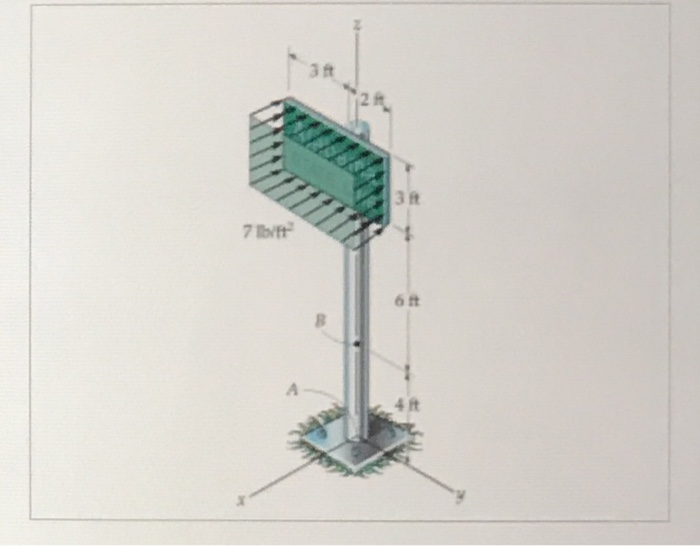Determine: -the resultant internal normal force acting on the cross section through point B of the signpost. The post is fixed to the ground and a uniform pressure of 7 lb/ft^2 acts perpendicular to the face of the sign. -the resultant internal shear force acting on the cross section through point B of the sign post. -the resultant internal bending moment acting on the cross section through point B of the signpost -the resultant internal torsional moment acting on the...

• ### Determine the normal force, shear force, and moment in the beam at sections passing through points D and E. Point E is just to the right of the 3k load.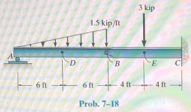Determine the normal force, shear force, and moment in the beam at sections passing through points D and E. Point E is just to the right of the 3k load.

• ### Consider the beam shown in (Figure 1). Suppose that M= 7 kip·ft, P = 4 kip, w = 1.1 kip/ft, and point D is located just to the left of the 4-kip load. Follow the sign convention.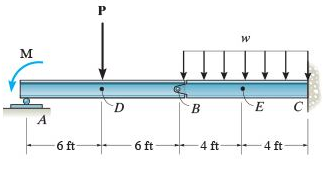Consider the beam shown in (Figure 1). Suppose that M= 7 kip·ft, P = 4 kip, w = 1.1 kip/ft, and point D is located just to the left of the 4-kip load. Follow the sign convention. Part A Determine the internal normal force at section passing through point D. Part B Determine the internal shear force at section passing through point D. Part C Determine the internal moment at section passing through point D. Part D Determine the internal normal force at section passing through point E. Part...

• ### 1) Find the normal force, shear force and bending moment at point C, along section c-c,...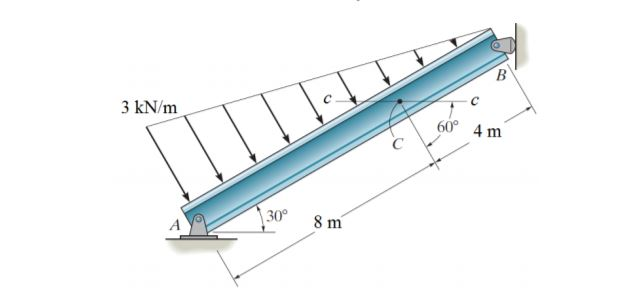1) Find the normal force, shear force and bending moment at point C, along section c-c, using lower segment. 2) Redo part (1) using upper segment. 3 kN/m 60° 4 m 30° 8 m

• ### A)Determine the internal normal force at point C, if F = 45 kN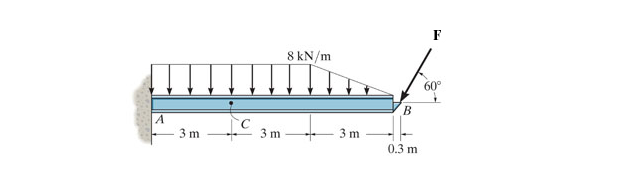A)Determine the internal normal force at point C, if F = 45 kNB)Determine the shear force at point C.C)Determine the bending moment at point C

• ### Determine the normal force, shear force, and moment at point C. Take that w = 4.2 kip/ft.(Figure 1)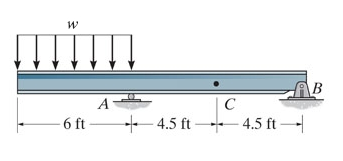Determine the normal force, shear force, and moment at point C. Take that w = 4.2 kip/ft.(Figure 1)Part A Determine the normal force at point C. Express your answer to three significant figures and include the appropriate units. Part B Determine the shear force at point C. Express your answer to three significant figures and include the appropriate units.  Part C Determine the moment at point C. Express your answer to three significant figures and include the appropriate units.

• ### Determine the internal normal force at point C. Take F1 = 467 lb , F2 = 165 Ib , F3 = 274 Ib.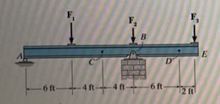Part A Determine the internal normal force at point C. Take F1 = 467 lb , F2 = 165 Ib , F3 = 274 Ib. (Figure 1) Part B Determine the shear force at point C. Part C Determine the moment at point C. Part D Determine the intemal normal force at point D. Part E Determine the shear force at point D. Part F Determine the moment at point D.

• ### Determine the normal force, shear force, and moment at point C.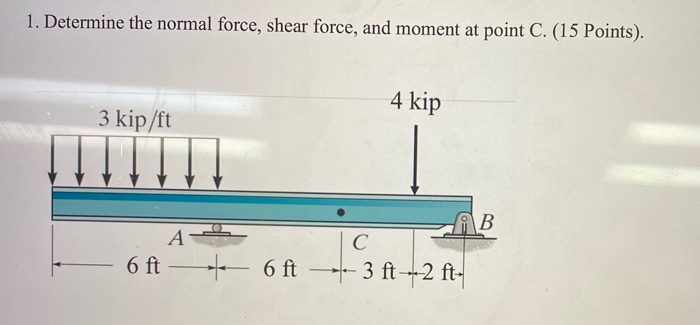Determine the normal force, shear force, and moment at point C.